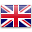# age 9 - 11

Sort by:
##### 3.1 物質的性質 Properties of Matter

STEM lessons + blocks

Students will gain understanding of how an object...##### 3.11 撇號 Apostrophe

STEM lessons + blocks

Students will learn how an apostrophe is used....##### 3.2 摩斯密碼 Morse Code

STEM lessons + blocks

Students will learn how Morse Code enabled efficient,...##### 3.3 結他 Guitar

STEM lessons + blocks

Students will gain understanding of how stringed instruments...##### 3.4 地震模擬器 Earthquake Simulator

STEM lessons + blocks

Students will gain understanding of what an earthquake...##### 3.5 火星探測號 Mars Rover

STEM lessons + blocks

Students will identify two possible solutions for designing...##### 3.6 燈塔 Lighthouse

STEM lessons + blocks

Students will investigate how light reflecting from objects...##### 3.7 信息的傳遞 SAM Insect

STEM lessons + blocks

Students will work collaboratively to investigate how information...##### 3.8 熔岩燈 SAM Lava Lamp

STEM lessons + blocks

Students will gain understanding of water erosion and...